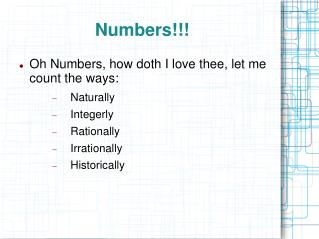DownloadDownload PresentationNumbers!!!

# Numbers!!!

Télécharger la présentation## Numbers!!!

- - - - - - - - - - - - - - - - - - - - - - - - - - - E N D - - - - - - - - - - - - - - - - - - - - - - - - - - -
##### Presentation Transcript

1. Numbers!!! • Oh Numbers, how doth I love thee, let me count the ways: • Naturally • Integerly • Rationally • Irrationally • Historically

2. Real Number SetsNatural Numbers • Natural Numbers are also known as “counting numbers.” • The set of natural numbers contains the numbers 0, 1, 2, 3, 4, … • Mathematically written as: N = {0, 1, 2, 3, 4, ...}

3. Real Number SetsIntegers • The set of integers include all natural numbers and their negatives. • The set of integers contains the numbers ...-4, -3, -2, -1, 0, 1, 2, 3, 4, … • Mathematically written as: Z = {…, -2, -1, 0, 1, 2, 3, 4, …} • Note: Z stands for Zahlen – German for “numbers.”

4. Real Number SetsRational Numbers • Rational Numbers are fractions (ratios). • The set of rational numbers contains the numbers written , where p and q are integers and q does not equal zero. • Mathematically written as: Q = { where p and q are integers, q≠0} • Note: Q stands for Quotient

5. Real Number SetsIrrational Numbers • The set of Irrational Numbers include all decimal numbers that neither terminate nor repeat. • The set of irrational numbers contains numbers like • Irrational Numbers cannot be written as a ratio between two numbers.

6. Real Number Sets • The set of real numbers include all previous sets of numbers. • Mathematically known as R.

7. History of Numbers • Today's numbers, also called Hindu-Arabic numbers, are a combination of just 10 symbols or digits: 1, 2, 3, 4, 5, 6, 7, 8, 9, and 0. These digits were introduced in Europe within the XII century by Leonardo Pisano (aka Fibonacci), an Italian mathematician. L. Pisano was educated in North Africa, where he learned and later carried to Italy the now popular Hindu-Arabic numerals. • Hindu numeral system is a pure place-value system, that is why you need a zero. Only the Hindus, within the context of Indo-European civilizations, have consistently used a zero. The Arabs, however, played an essential part in the dissemination of this numeral system.

8. History of Numbers • Numerals as they traveled from India to Europe

9. Maya Two Systems of Numbers • Left – 20 digit system • Above-anthropomorphic • system up to ten

10. Chinese Systems The Chinese use three numeral systems: the Hindu-Arabic numerals, along with two indigenous numeral systems, one for everyday writing (simple numerals), and another one for use in commercial or financial contexts (complex numerals). These last ones are used on checks and other transaction forms because they are much more difficult to alter. Actually, they are the equivalent of writing 'one', 'two', 'three', etc., rather than 1, 2, 3...

11. Greenlandic • Greenlanders use a twenty-base (vigesimal) system of numeration, divided into recurring five-based (quinary) periods of reckoning referring to the fingers and toes of a person! • When you run out of fingers and toes, you go on to the next person...and the next...and the next... • They call this “man counted out.”

12. Maori Counting • Maori are the indigenous people of New Zealand. They do something very interesting with numbers. If a number is for a thing, they prefix it with “ka”, if it is for a person, they prefix it with “toko.” • One = tahi, about one thing it is katahi, for a person it would be tokotahi.

13. Sumerian Numeration • The Sumerians, who lived around 3200 B.C. on the lower reaches of the river Euphrates. They had two systems of numeration: a sexagesimal system (base 60) for astronomical observation, and a decimal system for everyday use.

14. Sumerian Numeration • Building the numbers with two different size rods. • Then later with finer tools.

15. Sumerian Numeration • This is the cuneiform symbolic system.

16. Other Original Systems of Numeration • Other original systems of numeration were being used in the past. The "Notae Elegantissimae" shown below allow to write numbers from 1 to 9999. They are useful as a mnemotechnic aid, e.g. the symbol K in the example may mean 1414 (the first 4 figures of the square root of 2).

17. Do other people still think of numbers differently?